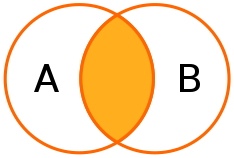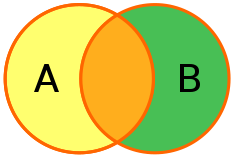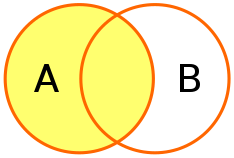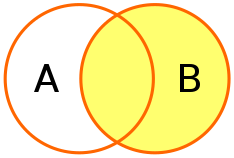# Merge

Merge function help us to merge two data frames by common columns or row names.

It is always best to explicitly state the identifiers on which you want to merge; it’s safer if the input data.frames change unexpectedly and easier to read later on. By using the merge function and its optional parameters you can specify by which variable you want to merge the frames to be sure that the matching is in the fields you desire.

Lets see now some examples of different merges.

We are going to create two simple data.frames:

``````dfA <- data.frame (TreeId = c(1:6), Specie = c(rep ("Pine", 3), rep ("Spruce", 3)))
dfB <- data.frame (TreeId = c(2, 4, 6), DevelopmentClass = c(rep ("Young", 2),
rep ("Old", 1)))

print(dfA)``````
``````##   TreeId Specie
## 1      1   Pine
## 2      2   Pine
## 3      3   Pine
## 4      4 Spruce
## 5      5 Spruce
## 6      6 Spruce``````
``print(dfB)``
``````##   TreeId DevelopmentClass
## 1      2            Young
## 2      4            Young
## 3      6              Old``````

## Inner mergeInner merge (Source: wikipedia)

An inner merge requires each row in the two merged tables to have matching column values. The inner merge will create a new table with those trees that have same ID number in A and B, it will compare each row of A with each row of B to find all pairs of rows that have same tree ID.

``merge(dfA, dfB, by = "TreeId") ``
``````##   TreeId Specie DevelopmentClass
## 1      2   Pine            Young
## 2      4 Spruce            Young
## 3      6 Spruce              Old``````

You can also use the by.x and by.y parameters if the matching variables have different names in the different data frames, for example:

``````dfC <- data.frame (TreeID = c(1:6), Specie = c(rep ("Pine", 3), rep ("Spruce", 3)))
dfD <- data.frame (TreeId = c(2, 4, 6), DevelopmentClass = c(rep ("Young", 2),                                                            rep ("Old", 1)))

print(dfC)``````
``````##   TreeID Specie
## 1      1   Pine
## 2      2   Pine
## 3      3   Pine
## 4      4 Spruce
## 5      5 Spruce
## 6      6 Spruce``````
``print(dfD)``
``````##   TreeId DevelopmentClass
## 1      2            Young
## 2      4            Young
## 3      6              Old``````
``merge(dfC, dfD, by.x = "TreeID", by.y = "TreeId") ``
``````##   TreeID Specie DevelopmentClass
## 1      2   Pine            Young
## 2      4 Spruce            Young
## 3      6 Spruce              Old``````

## Outer merge

An Outer merge could be a full outer merge, a left outer merge or right outer merge.

### Full outer mergeFull outer merge (Source: wikipedia)

A full outer merge combines the effect of applying both left and right outer merges. In this case we will have all rows from both tables, including those that do not match, in those unmatching cases we will have NULL value for the new common variables:

``merge(x = dfA, y = dfB, by = "TreeId", all = TRUE)``
``````##   TreeId Specie DevelopmentClass
## 1      1   Pine             <NA>
## 2      2   Pine            Young
## 3      3   Pine             <NA>
## 4      4 Spruce            Young
## 5      5 Spruce             <NA>
## 6      6 Spruce              Old``````

### Left outer mergeLeft outer merge (Source: wikipedia)

A left outer merge for tables A and B always contains all rows of the “left” table (A), even if the merge-condition does not find any matching row in the “right” table (B). Here we will also have NULL values in rows (TreeIds) that are in A but not in B.

``merge(x = dfA, y = dfB, by = "TreeId", all.x = TRUE)``
``````##   TreeId Specie DevelopmentClass
## 1      1   Pine             <NA>
## 2      2   Pine            Young
## 3      3   Pine             <NA>
## 4      4 Spruce            Young
## 5      5 Spruce             <NA>
## 6      6 Spruce              Old``````

### Right outer mergeLeft outer merge (Source: wikipedia)

A right outer merge for tables A and B always contains all rows of the “right” table (B), even if the merge-condition does not find any matching row in the “left” table (A). Here we will also have NULL values in rows (TreeIds) that are in B but not in A.

``merge(x = dfA, y = dfB, by = "TreeId", all.y = TRUE)``
``````##   TreeId Specie DevelopmentClass
## 1      2   Pine            Young
## 2      4 Spruce            Young
## 3      6 Spruce              Old``````